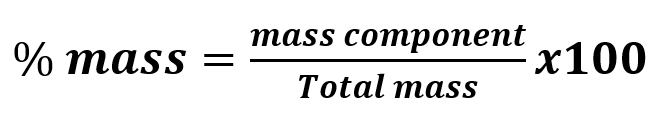# Problem: Copper can be drawn into thin wires. How many meters of 34-gauge wire (diameter = 6.304 x 10−3 in) can be produced from the copper in 5.01 lb of covellite, an ore of copper that is 66% copper by mass? (Hint: Treat the wire as a cylinder: V of cylinder = πr2h; d of copper = 8.95 g/cm3.)

###### FREE Expert Solution

First, we have to notice that the mass given was for an impure substance containing copper. This means that we have to use mass % to calculate the mass of copper.Apply the formula to the problem then rearrange to isolate mass of Cu (Copper)###### Problem Details

Copper can be drawn into thin wires. How many meters of 34-gauge wire (diameter = 6.304 x 10−3 in) can be produced from the copper in 5.01 lb of covellite, an ore of copper that is 66% copper by mass? (Hint: Treat the wire as a cylinder: V of cylinder = πr2h; d of copper = 8.95 g/cm3.)